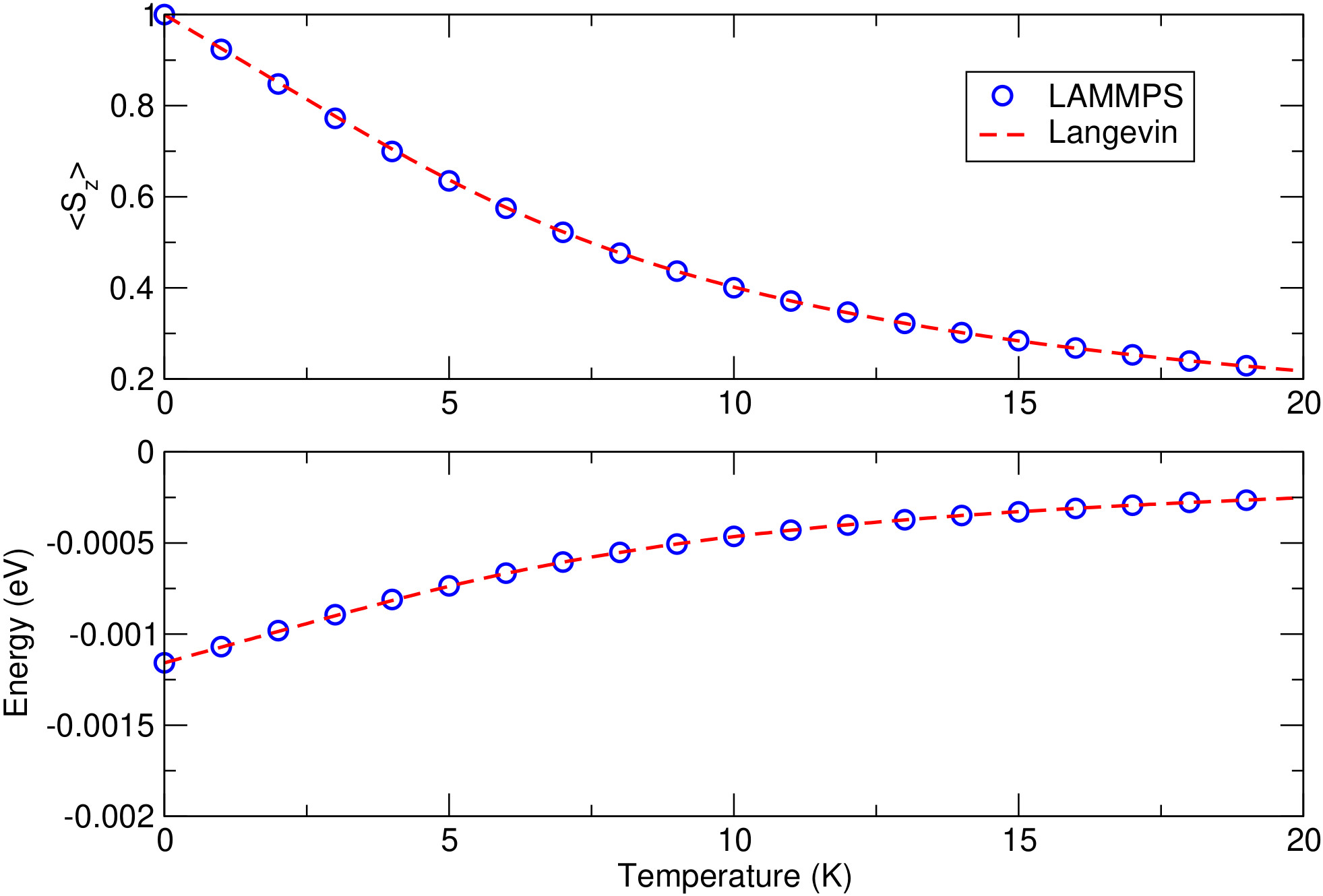$$\renewcommand{\AA}{\text{Å}}$$

# fix precession/spin command

## Syntax

fix ID group precession/spin style args

• ID, group are documented in fix command

• precession/spin = style name of this fix command

• style = zeeman or anisotropy or cubic or stt

zeeman args = H x y z
H = intensity of the magnetic field (in Tesla)
x y z = vector direction of the field
anisotropy args = K x y z
K = intensity of the magnetic anisotropy (in eV)
x y z = vector direction of the anisotropy
cubic args = K1 K2c n1x n1y n1x n2x n2y n2z n3x n3y n3z
K1 and K2c = intensity of the magnetic anisotropy (in eV)
n1x to n3z = three direction vectors of the cubic anisotropy
stt args = J x y z
J = intensity of the spin-transfer torque field
x y z = vector direction of the field

## Examples

fix 1 all precession/spin zeeman 0.1 0.0 0.0 1.0
fix 1 3 precession/spin anisotropy 0.001 0.0 0.0 1.0
fix 1 iron precession/spin cubic 0.001 0.0005 1.0 0.0 0.0 0.0 1.0 0.0 0.0 0.0 1.0
fix 1 all precession/spin zeeman 0.1 0.0 0.0 1.0 anisotropy 0.001 0.0 0.0 1.0


## Description

This fix applies a precession torque to each magnetic spin in the group.

Style zeeman is used for the simulation of the interaction between the magnetic spins in the defined group and an external magnetic field:

$H_{Zeeman} = -g \sum_{i=0}^{N}\mu_{i}\, \vec{s}_{i} \cdot\vec{B}_{ext}$

with:

• $$\vec{B}_{ext}$$ the external magnetic field (in T)

• $$g$$ the Lande factor (hard-coded as $$g=2.0$$)

• $$\vec{s}_i$$ the unitary vector describing the orientation of spin $$i$$

• $$\mu_i$$ the atomic moment of spin $$i$$ given as a multiple of the Bohr magneton $$\mu_B$$ (for example, $$\mu_i \approx 2.2$$ in bulk iron).

The field value in Tesla is multiplied by the gyromagnetic ratio, $$g \cdot \mu_B/\hbar$$, converting it into a precession frequency in rad.THz (in metal units and with $$\mu_B = 5.788\cdot 10^{-5}$$ eV/T).

As a comparison, the figure below displays the simulation of a single spin (of norm $$\mu_i = 1.0$$) submitted to an external magnetic field of $$\vert B_{ext}\vert = 10.0\; \mathrm{Tesla}$$ (and oriented along the z axis). The upper plot shows the average magnetization along the external magnetic field axis and the lower plot the Zeeman energy, both as a function of temperature. The reference result is provided by the plot of the Langevin function for the same parameters.The temperature effects are accounted for by connecting the spin $$i$$ to a thermal bath using a Langevin thermostat (see fix langevin/spin for the definition of this thermostat).

Style anisotropy is used to simulate an easy axis or an easy plane for the magnetic spins in the defined group:

$H_{aniso} = -\sum_{{ i}=1}^{N} K_{an}(\mathbf{r}_{i})\, \left( \vec{s}_{i} \cdot \vec{n}_{i} \right)^2$

with $$n$$ defining the direction of the anisotropy, and $$K$$ (in eV) its intensity. If $$K > 0$$, an easy axis is defined, and if $$K < 0$$, an easy plane is defined.

Style cubic is used to simulate a cubic anisotropy, with three possible easy axis for the magnetic spins in the defined group:

$H_{cubic} = -\sum_{{ i}=1}^{N} K_{1} \Big[ \left(\vec{s}_{i} \cdot \vec{n}_1 \right)^2 \left(\vec{s}_{i} \cdot \vec{n}_2 \right)^2 + \left(\vec{s}_{i} \cdot \vec{n}_2 \right)^2 \left(\vec{s}_{i} \cdot \vec{n}_3 \right)^2 + \left(\vec{s}_{i} \cdot \vec{n}_1 \right)^2 \left(\vec{s}_{i} \cdot \vec{n}_3 \right)^2 \Big] +K_{2}^{(c)} \left(\vec{s}_{i} \cdot \vec{n}_1 \right)^2 \left(\vec{s}_{i} \cdot \vec{n}_2 \right)^2 \left(\vec{s}_{i} \cdot \vec{n}_3 \right)^2$

with $$K_1$$ and $$K_{2c}$$ (in eV) the intensity coefficients and $$\vec{n}_1$$, $$\vec{n}_2$$ and $$\vec{n}_3$$ defining the three anisotropic directions defined by the command (from n1x to n3z). For $$\vec{n}_1 = (1 0 0)$$, $$\vec{n}_2 = (0 1 0)$$, and $$\vec{n}_3 = (0 0 1)$$, $$K_1 < 0$$ defines an iron type anisotropy (easy axis along the $$(0 0 1)$$-type cube edges), and $$K_1 > 0$$ defines a nickel type anisotropy (easy axis along the $$(1 1 1)$$-type cube diagonals). $$K_2^c > 0$$ also defines easy axis along the $$(1 1 1)$$-type cube diagonals. See chapter 2 of (Skomski) for more details on cubic anisotropies.

Style stt is used to simulate the interaction between the spins and a spin-transfer torque. See equation (7) of (Chirac) for more details about the implemented spin-transfer torque term.

In all cases, the choice of $$(x y z)$$ only imposes the vector directions for the forces. Only the direction of the vector is important; its length is ignored (the entered vectors are normalized).

Those styles can be combined within one single command line.

Note

The norm of all vectors defined with the precession/spin command have to be non-zero. For example, defining “fix 1 all precession/spin zeeman 0.1 0.0 0.0 0.0” would result in an error message. Since those vector components are used to compute the inverse of the field (or anisotropy) vector norm, setting a zero-vector would result in a division by zero.

## Restart, fix_modify, output, run start/stop, minimize info

The fix_modify energy option is supported by this fix to add the energy associated with the spin precession torque to the global potential energy of the system as part of thermodynamic output. The default setting for this fix is fix_modify energy no.

This fix computes a global scalar which can be accessed by various output commands. The scalar is the potential energy (in energy units) discussed in the previous paragraph. The scalar value is an “extensive” quantity.